Next: B. Ray-Isosurface Intersection Up: Dissertation Otmar Ertl Previous: 7. Summary and Outlook

# A. Line-Triangle Intersection

For the initialization of the LS function, triangles must be checked for possible intersections with grid lines (see Section 4.1.1). In the following, a robust line-triangle intersection test is presented. If the line intersects the triangle, the intersection point is also calculated. The following approach ensures that, if a line close to an edge fails the intersection test due to numerical errors, the test will succeed for another triangle, which is adjacent to the same edge. Hence, if a surface is given as a triangulation, cases, where the line fails all intersection tests, although it intersects the surface, are avoided.

A triangle with vertices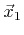,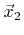, and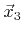is intersected by a line defined by point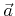and unit vector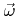, if the (signed) areas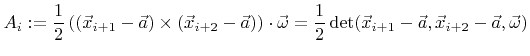(A.1)

are either all non-negative or all non-positive. The sign is given by the triangle orientation with respect to. All the index summations in (A.1) are modulo 3 plus 1.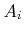corresponds to the area of the triangle spanned by the vectors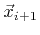,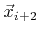, and, if it is projected onto a plane perpendicular to(see Figure A.1). If allare equal to zero, the grid line is in the same plane as the triangle, or the triangle is degenerated. In both cases the line is considered to not intersect the triangle.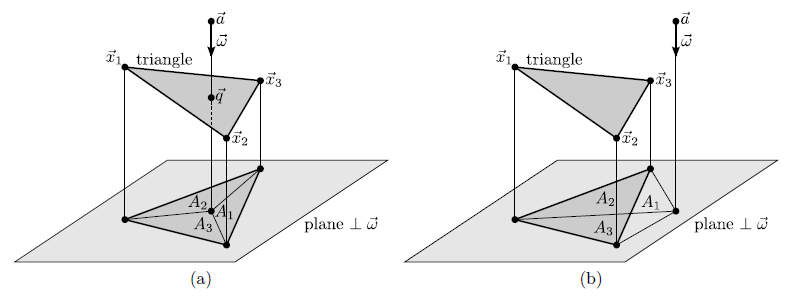To guarantee that lines close to an edge succeed the intersection test for any of the two adjacent triangles, the numerical evaluation of (A.1) must preserve the anticommutativity with respect toand. However, due to numerical errors this is usually not the case. For example, the numerical result of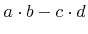is not necessarily the same as that of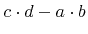with opposite sign . To overcome this problem, the two vectorsandin (A.1) are compared using an order relation, such as lexicographical comparison. Ifis larger than, the two vectors are swapped, and the final result of (A.1) is inverted. This technique ensures the anticommutativity in (A.1).

Once the areasare determined and the intersection test is positive, the intersection point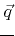can be obtained by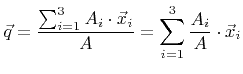with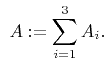(A.2)

As defined earlier, an intersection only occurs, if allare non-negative or non-positive, while not all are equal to zero. Therefore, the denominator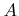in (A.2) never vanishes. To avoid potential overflows the second variant for the calculation of the intersection point is preferable. There, the coefficients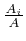are always in the range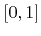and the calculation of the intersection point is safe.Next: B. Ray-Isosurface Intersection Up: Dissertation Otmar Ertl Previous: 7. Summary and Outlook

Otmar Ertl: Numerical Methods for Topography Simulation